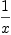login  home  contents  what's new  discussion  bug reports help  links  subscribe  changes  refresh  edit

# Edit detail for Where do variables belong? revision 3 of 3

 1 2 3 Editor: test1 Time: 2019/07/20 19:59:52 GMT+0 Note:

changed:
-Currently, Axiom allows
Currently, FriCAS allows

changed:
-I think that this is calling for trouble, see issue #270. There are two ways to fix this:
Bill Page thinks that this is calling for trouble, see issue #270. There are two ways to fix this:

changed:
-- make the coercion to 'UP(x, EXPR INT)' and the like smarter, allowing only coefficients that do not contain the variable 'x'. This is what I'm going to discuss on this page.
-
-More precisely: For any polynomial (or series) domain that has a specified list of variables, I want that axiom ensures that any coefficient of a member does not contain one of these variables. Examples::
- make the coercion to 'UP(x, EXPR INT)' and the like smarter, allowing only coefficients that do not contain the variable 'x'. This is what is discussed on this page.

More precisely: For any polynomial (or series) domain that has a specified list of variables, I (Bill Page) want that
FriCAS ensures that any coefficient of a member does not contain one of these variables. Examples::


Currently, FriCAS allows

fricas
(1/x)::UP(x, FRAC POLY INT)(1)
Type: UnivariatePolynomial(x,Fraction(Polynomial(Integer)))

and

fricas
(1/x)::UP(x, EXPR INT)(2)
Type: UnivariatePolynomial(x,Expression(Integer))

Bill Page thinks that this is calling for trouble, see issue #270. There are two ways to fix this:

• forbid (or rather depreciate) towering of domains that contain variables

This is probably the easier way out. However, in order to allow polynomials like sin(2)*x + sin(3)*x^2, new domains UEXPR and MEXPR paralleling UP and MPOLY would be needed. These are on the OldWishList...

• make the coercion to UP(x, EXPR INT) and the like smarter, allowing only coefficients that do not contain the variable x. This is what is discussed on this page.

More precisely: For any polynomial (or series) domain that has a specified list of variables, I (Bill Page) want that FriCAS ensures that any coefficient of a member does not contain one of these variables. Examples:

 (1/x)::UP(x, EXPR INT)
x*2^x::UP(x, EXPR INT)
(1/x)::UP(x, FRAC POLY INT)
(1/x)::UTS(FRAC POLY INT,x,0)


all produce an error Coefficient contains variable. However, for example:

 x*2^y::UP(x, EXPR INT)


would be ok. More generally, the "outermost" domain should "take" all the variables: POLY EXPR INT would be the domain of polynomials with coefficients that do not contain any variables. For example, (x*sin(2))::POLY EXPR INT would be ok, (x*sin(y))::POLY EXPR INT would produce an error. In this setting, the domain EXPR POLY INT would be the same as EXPR INT.

To achieve this, we need to be able to test wether a given element of a given ring contains a specific variable. Unfortunately, this is not as easy as it seems. For example, FRAC POLY INT does not have a function variables. Rather, this function is provided by RF. The reason is, that the values of variables are in VarSet. For example SUP has as variable the unique element in SAOS. This raises another question, discussed in http://lists.gnu.org/archive/html/axiom-developer/2004-09/msg00021.html a (partial) solution to which I noted in http://lists.gnu.org/archive/html/axiom-developer/2004-09/msg00044.html

However for our purposes something rather primitive is sufficient: All domains that contain variables need to export a function variables2: % -> List Symbol. Then we can say in 'QFCAT':

 if S has variables2: S -> List Symbol then
variables2: % -> List Symbol
variables2 f ==
merge(variables2(numer(f)), variables2(denom(f)))


and add the following to UP and 'MPOLY':

 if R has variables2: R -> List Symbol then
coerce(r:R):% ==
if member?(x, variables2(r)) then
error "coefficient contains variable"
else coerce(r)\$Rep